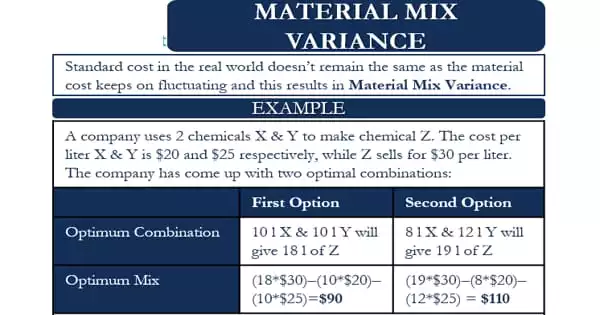Accounting

# Concept of Material Mix Variance (MMV)The material mix variance is caused by a divergence in the actual composition of a material mixture from the standard one. The difference between the budgeted and actual mixes of direct material costs employed in a production process is referred to as direct material mix variation. A material mix variance occurs when the composition of a material mixture changes, which occurs when more than one type of material is utilized in a definite proportion.

In such a case, the material variance is determined independently for each material type. The variance can be used to determine whether a lower-cost material mix can be employed to make a product. The notion is only useful when it is possible to change the material mix without lowering the quality of the resulting product below a certain threshold.

The material mix variance is calculated by using the following formula:

Material Mix Variance(MMV) = SP x (RSQ-AQ)

Where,

• SP = Standard Price
• RSQ = Revised standard quantity
• AQ = Actual Quantity

Material Mix Variance is a term used in the manufacturing industry. Manufacturing companies invest a lot of money on R&D to figure out the best material blend. This ideal blend is a mixture of components that reduces production costs while retaining desired quality. Companies utilize this optimal material combination as a standard to define their cost and budgetary estimates.

However, in the real world, this standard cost does not remain constant because material costs fluctuate. As a result, the cost differs from the typical cost. This is referred to as Material Mix Variance. The variation might also be attributed to a change in quantity from the optimum combination. We can state that the variance occurs as a result of a change in the ratio in which a corporation employs the materials in contrast to the established norm.

Example of Material Mix Variance

An example will help us understand the concept in a better way.  A company uses two chemicals X and Y to make a third chemical Z. The cost per liter of X and Y is \$20 and \$25, respectively, while Z sells for \$30 per liter.

The company, on the basis of its research, has come up with two optimal combinations. These are:

First: 10 ltr. of X and 10 ltr. of Y will give 18 ltr. of Z

Second: 8 ltr. of X and 12 ltr. of Y will give 19 ltr. of Z

We can determine the optimum mix between the two by calculating the net contribution from each.

First: (18 x \$30) less (10 x \$20) less (10 x \$25) = \$90

Second: (19 x \$30) less (8 x \$20) less (12 x \$25) = \$110

This suggests that the second mix is the best blend because it provides the greatest net contribution. Now, if the prices of X and Y fluctuate, or the selling price of Z changes, or if the production uses more materials than the ideal mix, the variance will occur.

Assume the corporation makes 1,900 ltr. of Z from 900 ltr. of X and 1,100 ltr. of Y. X and Y are the same price as the usual cost above, i.e. \$20 and \$25, respectively. To compute the variance, we must first determine the standard cost of generating 1,850 ltr. of Z using the proportionately optimum mix of X and Y from the Second combination above.

The optimum quantity of X, in this case, will be = (8/20)* 2,000 ltr. = 800 ltr. The 2,000 ltr. here is the total X and Y used to produce Z. Similarly, the optimum quantity of Y will be = (12/20)* 2,000 ltr. = 1,200 ltr.